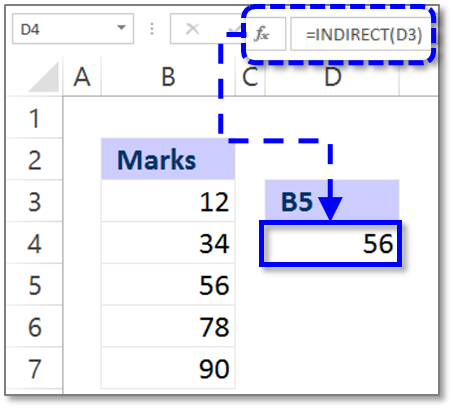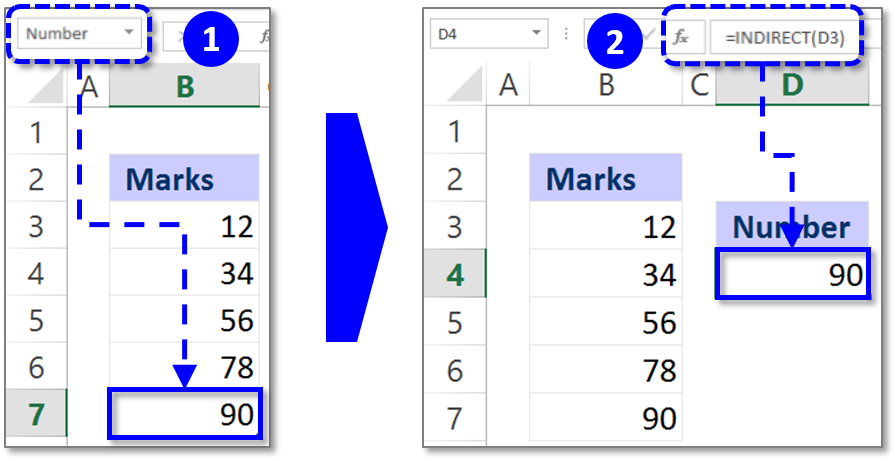Need Help? Chat with us

# Indirect function in Excel

## Indirect function in Excel with an example

The tutorial will explain you about the Excel Indirect function. How they are used and what is the Syntax of it?

The Excel Indirect function returns the reference which is specified to a cell or text string. The resulting answer will be the content displayed in that cell for which the reference is provided. The Indirect function is an inbuilt function in Excel under the head Lookup/Reference function.

## Special Offer for First Time Users

If you are the first time user and want to learn Microsoft office skills, then you can avail this special offer discount on your email.It can be used as a worksheet function in Excel and also it can be entered as a part of the formula. The Indirect function was introduced in the year 2000. The Excel Indirect function does not perform any calculation nor does it test conditions or logical test.

NOTE: The indirect reference won’t change even if new rows or columns are added or subtracted in the worksheet.

It is easier to understand but let us now see how it works in Excel by first going through the Syntax and then with the various examples.

Syntax of Indirect Function:

INDIRECT (ref_text, [a1])

There are two arguments in the Excel Indirect function. One is compulsory and the other is optional.

Ref_text: The ref­_text is the compulsory argument that needs to be entered. It is the reference that a cell contains as a text.

A1: The a1 is the optional argument that the reference can be an A1 style reference, R1C1 style reference or a name defined reference. If the reference is not valid it will return #REF! value.

NOTE: Indirect function is useful when we want to convert a text value into a valid cell reference.

The default setting is True i.e. The A1 reference style and the False is R1C1 reference style.

POINTS TO REMEMBER:

1. The indirect function is a volatile function that means it needs to be used cautiously.

2. When the ref­_text is in the workbook that workbook needs to be open at the time of using the indirect function in Excel or else it will return #REF! Error.

3.  A very rare used formula but immensely benefits when you start using it in the combination of other functions.

Example1: Using Reference cell to fetch the value

In the first example, we have a list of marks and we want to know the marks for a particular cell in the worksheet.

Now, will we use the indirect function?

First, we will select any cell in the worksheet and enter the cell reference. In our example, we have entered B5 in cell D3.

Now we enter the Indirect formula in the cell D4 as

=INDIRECT (D3)

Press Enter

Result: 56. We get the answer when we took the reference cell and it returned the value of that reference cell.But, if we would have entered double quotation before and after the cell reference it would return the same value as that of the cell we took reference off.

Also, note that if you insert or delete a row ahead of the resulting cell or above the cell then the result value will change accordingly.

Example 2: Using the reference name of the cell to find the value.

In the second example, we will give a name to the cell using the name box. We assigned Number as the name to the cell B7.

Now we will see how the indirect function works when we assigned the name to the cell.

In the cell, D3 enter the name assigned to the cell.

Now use the Indirect function

=INDIRECT(D3)

Press Enter

It will return 90 as the answer because the value of the reference name is 90.Conclusion: The INDIRECT function when used along with other Excel function will give a better result. The Indirect function can be used to calculate SUM, AVERAGE, MIN, MAX, MATCH, VLOOKUP and many more. This was a quick tutorial on how INDIRECT function works and how much is it capable for.

Hope you like our tutorial for more cool and amazing trick of excel Like our Excel Superstar Facebook Page and subscribe to our Excel Superstar YouTube channel. Excel Superstar is the leading online training company, which provides Online Excel Course in Hindi. Connect with us and become an Excel Superstar.